1601560800

# Autoencoders for Dimensionality Reduction

In the previous post, we explained how we can reduce the dimensions by applying PCA and t-SNE and how we can apply Non-Negative Matrix Factorization for the same scope. In this post, we will provide a concrete example of how we can apply Autoeconders for Dimensionality Reduction. We will work with Python and TensorFlow 2.x.

## Autoencoders on MNIST Dataset

We will use the MNIST dataset of tensorflow, where the images are 28 x 28 dimensions, in other words, if we flatten the dimensions, we are dealing with 784 dimensions. Our goal is to reduce the dimensions, from 784 to 2, by including as much information as possible.

Let’s get our hands dirty!

#autoencoder #dimensionality-reduction #data-science #data-visualization #tensorflow

## Buddha Community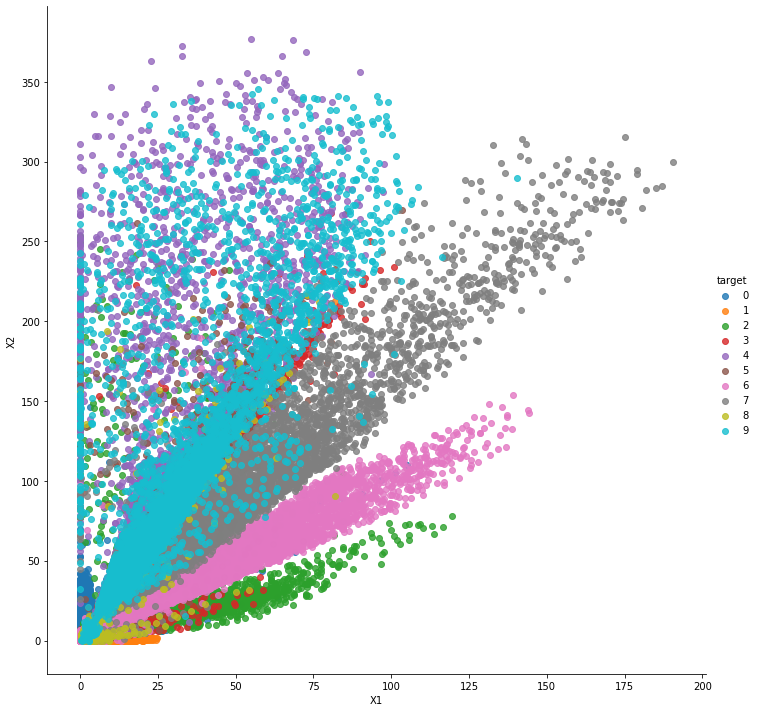1602817200

## Autoencoders for Dimensionality Reduction

In the previous post, we explained how we can reduce the dimensions by applying PCA and t-SNE and how we can apply Non-Negative Matrix Factorization for the same scope. In this post, we will provide a concrete example of how we can apply Autoeconders for Dimensionality Reduction. We will work with Python and TensorFlow 2.x.

## Autoencoders on MNIST Dataset

We will use the MNIST dataset of TensorFlow, where the images are 28 x 28 dimensions, in other words, if we flatten the dimensions, we are dealing with 784 dimensions. Our goal is to reduce the dimensions, from 784 to 2, by including as much information as possible.

Let’s get our hands dirty!

``````from tensorflow.keras.models import Sequential
from tensorflow.keras.layers import Dense,Flatten,Reshape
from tensorflow.keras.optimizers import SGD
from tensorflow.keras.datasets import mnist
(X_train, y_train), (X_test, y_test) = mnist.load_data()
X_train = X_train/255.0 X_test = X_test/255.0
#### Encoder
encoder = Sequential()
#### Decoder
decoder = Sequential()
#### Autoencoder
autoencoder = Sequential([encoder,decoder]) autoencoder.compile(loss="mse") autoencoder.fit(X_train,X_train,epochs=50)
encoded_2dim = encoder.predict(X_train)
## The 2D
AE = pd.DataFrame(encoded_2dim, columns = ['X1', 'X2'])
AE['target'] = y_train
sns.lmplot(x='X1', y='X2', data=AE, hue='target', fit_reg=False, size=10)
``````

#autoencoder #dimensionality-reduction #data-science #data-visualization #tensorflow1601560800

## Autoencoders for Dimensionality Reduction

In the previous post, we explained how we can reduce the dimensions by applying PCA and t-SNE and how we can apply Non-Negative Matrix Factorization for the same scope. In this post, we will provide a concrete example of how we can apply Autoeconders for Dimensionality Reduction. We will work with Python and TensorFlow 2.x.

## Autoencoders on MNIST Dataset

We will use the MNIST dataset of tensorflow, where the images are 28 x 28 dimensions, in other words, if we flatten the dimensions, we are dealing with 784 dimensions. Our goal is to reduce the dimensions, from 784 to 2, by including as much information as possible.

Let’s get our hands dirty!

#autoencoder #dimensionality-reduction #data-science #data-visualization #tensorflow1619766480

## Introduction

Principal Component Analysis (PCA) is one of the most popular dimensionality reduction algorithms. PCA works by finding the axes that account for the larges amount of variance in the data which are orthogonal to each other. The iᵗʰ axis is called the iᵗʰ principal component (PC). The steps to perform PCA are:

• Standardize the data.
• Obtain the Eigenvectors and Eigenvalues from the covariance matrix or correlation matrix, or perform Singular Value Decomposition.

We will perform PCA with the implementation of sklearn: it uses Singular Value Decomposition (SVD) from scipy (scipy.linalg). SVD is a factorization of a 2D matrix A. it is written as: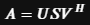S is a 1D array which contains the singular values of AU and V^H are unitary (UU = UUᵗ = I). Most PCA implementation performs SVD to improve computational efficiency.

An Autoencoder (AE) on the other hand is a special kind of neural network which is trained to copy its input to its output. First, it maps the input to a latent space of reduced dimension, then code back the latent representation to the output. An AE learns to compress data by reducing the reconstruction error.

We will see in a moment how to implement and compare both PCA and Autoencoder results.

We will generate our data with make_classification from sklearn which will also give us some labels. We will use those labels to make comparisons between the efficiency of the clustering between our methods.

There will be three sections.

In the first we will implement a simple undercomplete linear autoencoder: that is, an autoencoder with a single layer which is of lower dimension than its input. The second section will introduce a Stacked Linear Autoencoder.

In the third section, we will try to modify the activation functions to address more complexity.

The results will be compared graphically with a PCA and in the end we will try to predict the classes using a simple random forest classification algorithm with cross validation.

#neural-networks #dimensionality-reduction #deep-learning #tensorflow #python1597741140

## Dimensionality Reduction

It is easy for us to visualize two or three dimensional data, but once it goes beyond three dimensions, it becomes much harder to see what high dimensional data looks like.

Today we are often in a situation that we need to analyze and find patterns on datasets with thousands or even millions of dimensions, which makes visualization a bit of a challenge. However, a tool that can definitely help us better understand the data is dimensionality reduction.

In this post, I will discuss t-SNE, a popular non-linear dimensionality reduction technique and how to implement it in Python using sklearn. The dataset I have chosen here is the popular MNIST dataset.

## Overview

T-Distributed Stochastic Neighbor Embedding, or t-SNE, is a machine learning algorithm and it is often used to embedding high dimensional data in a low dimensional space .

In simple terms, the approach of t-SNE can be broken down into two steps. The first step is to represent the high dimensional data by constructing a probability distribution P, where the probability of similar points being picked is high, whereas the probability of dissimilar points being picked is low. The second step is to create a low dimensional space with another probability distribution Q that preserves the property of P as close as possible.

In step 1, we compute the similarity between two data points using a conditional probability p. For example, the conditional probability of j given i represents that x_j would be picked by x_i as its neighbor assuming neighbors are picked in proportion to their probability density under a Gaussian distribution centered at _x_i _. In step 2, we let _y_i _and y_j to be the low dimensional counterparts of x_i and _x_j, _respectively. Then we consider q to be a similar conditional probability for y_j being picked by y_i and we employ a **student t-distribution **in the low dimension map. The locations of the low dimensional data points are determined by minimizing the Kullback–Leibler divergence of probability distribution P from Q.

#data-visualization #sklearn #dimensionality-reduction #data-science #machine-learning #data analysis1593271874

## Dimensionality Reduction in Python

This is a memo to share what I have learnt in Dimensionality Reduction in Python, capturing the learning objectives as well as my personal notes. The course is taught by Jerone Boeye, and it includes 4 chapters:

Chapter 1. Exploring high dimensional data

Chapter 2. Feature selection I, selecting for feature information

Chapter 3. Feature selection II, selecting for model accuracy

Chapter 4. Feature extractionPhoto by Aditya Chinchure on Unsplash

High-dimensional datasets can be overwhelming and leave you not knowing where to start. Typically, you’d visually explore a new dataset first, but when you have too many dimensions the classical approaches will seem insufficient. Fortunately, there are visualization techniques designed specifically for high dimensional data and you’ll be introduced to these in this course.

After exploring the data, you’ll often find that many features hold little information because they don’t show any variance or because they are duplicates of other features. You’ll learn how to detect these features and drop them from the dataset so that you can focus on the informative ones. In a next step, you might want to build a model on these features, and it may turn out that some don’t have any effect on the thing you’re trying to predict. You’ll learn how to detect and drop these irrelevant features too, in order to reduce dimensionality and thus complexity.

Finally, you’ll learn how feature extraction techniques can reduce dimensionality for you through the calculation of uncorrelated principal components.

## Chapter 1. Exploring high dimensional data

You’ll be introduced to the concept of dimensionality reduction and will learn when an why this is important. You’ll learn the difference between feature selection and feature extraction and will apply both techniques for data exploration. The chapter ends with a lesson on t-SNE, a powerful feature extraction technique that will allow you to visualize a high-dimensional dataset.

## Introduction

Dataset with more than 10 columns are considered high dimensional data.

## Finding the number of dimensions in a dataset

A larger sample of the Pokemon dataset has been loaded for you as the Pandas dataframe `pokemon_df`.

How many dimensions, or columns are in this dataset?

Answer: 7 dimensions, each Pokemon is described by 7 features.

``````In : pokemon_df.shape
Out: (160, 7)
``````

## Removing features without variance

A sample of the Pokemon dataset has been loaded as `pokemon_df`. To get an idea of which features have little variance you should use the IPython Shell to calculate summary statistics on this sample. Then adjust the code to create a smaller, easier to understand, dataset.

For the number_cols, Generation column has ‘1’ in all 160 rows.

``````In : pokemon_df.describe()
Out:
HP     Attack     Defense  Generation
count  160.00000  160.00000  160.000000       160.0
mean    64.61250   74.98125   70.175000         1.0
std     27.92127   29.18009   28.883533         0.0
min     10.00000    5.00000    5.000000         1.0
25%     45.00000   52.00000   50.000000         1.0
50%     60.00000   71.00000   65.000000         1.0
75%     80.00000   95.00000   85.000000         1.0
max    250.00000  155.00000  180.000000         1.0

# Remove the feature without variance from this list
number_cols = ['HP', 'Attack', 'Defense']
``````

For the non_number_cols, Legendary column has ‘False’ in all 160 rows.

``````In : pokemon_df[['Name', 'Type', 'Legendary']].describe()
Out:
Name   Type Legendary
count    160    160       160
unique   160     15         1
top     Abra  Water     False
freq       1     31       160

# Remove the feature without variance from this list
non_number_cols = ['Name', 'Type']
# Create a new dataframe by subselecting the chosen features
df_selected = pokemon_df[number_cols + non_number_cols]
# Prints the first 5 lines of the new dataframe
<script.py> output:
HP  Attack  Defense                   Name   Type
0  45      49       49              Bulbasaur  Grass
1  60      62       63                Ivysaur  Grass
2  80      82       83               Venusaur  Grass
3  80     100      123  VenusaurMega Venusaur  Grass
4  39      52       43             Charmander   Fire
``````

All Pokemon in this dataset are non-legendary and from generation one so you could choose to drop those two features.

## Feature selection vs feature extraction

Why reduce dimensionality?

· dataset will be less complex

· dataset will take up less storage space

· dataset will require less computation time

· dataset will have lower chance of model overfitting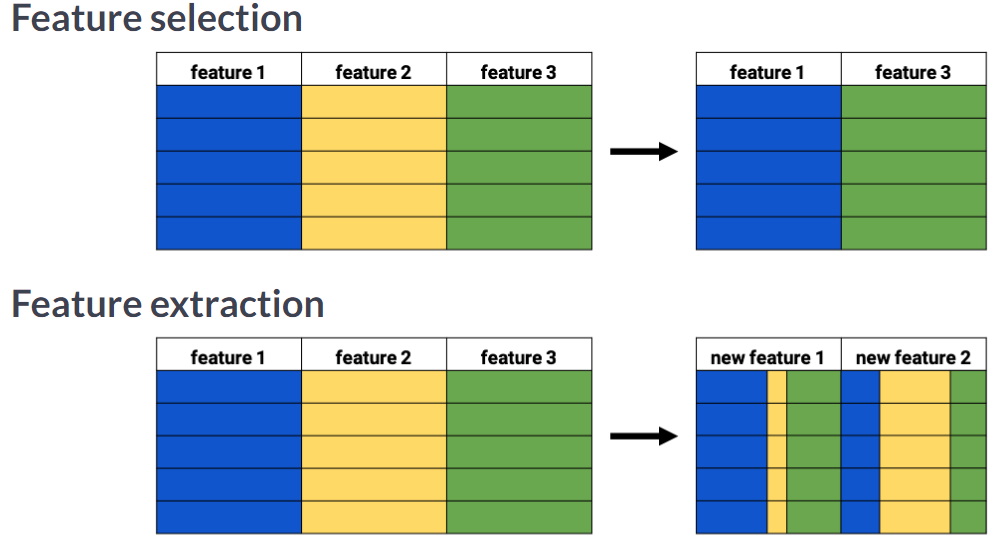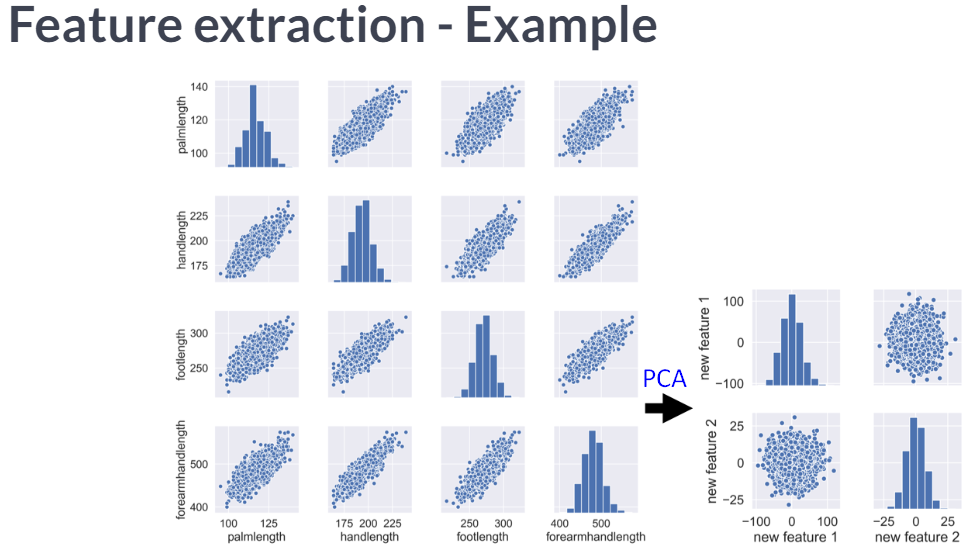## Visually detecting redundant features

Data visualization is a crucial step in any data exploration. Let’s use Seaborn to explore some samples of the US Army ANSUR body measurement dataset.

Two data samples have been pre-loaded as `ansur_df_1` and `ansur_df_2`.

Seaborn has been imported as `sns`.

``````# Create a pairplot and color the points using the 'Gender' feature
sns.pairplot(ansur_df_1, hue='Gender', diag_kind='hist')
plt.show()
``````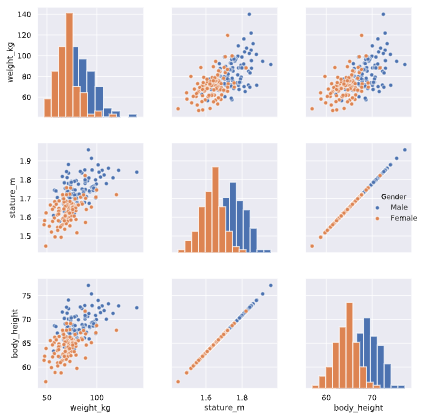Two features are basically duplicates, remove one of them from the dataset.

``````# Remove one of the redundant features
reduced_df = ansur_df_1.drop('stature_m', axis=1)

# Create a pairplot and color the points using the 'Gender' feature
sns.pairplot(reduced_df, hue='Gender')
# Show the plot
plt.show()
``````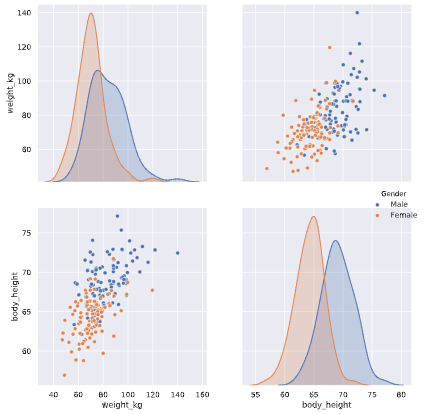``````# Create a pairplot and color the points using the 'Gender' feature
sns.pairplot(ansur_df_2, hue='Gender', diag_kind='hist')
plt.show()
``````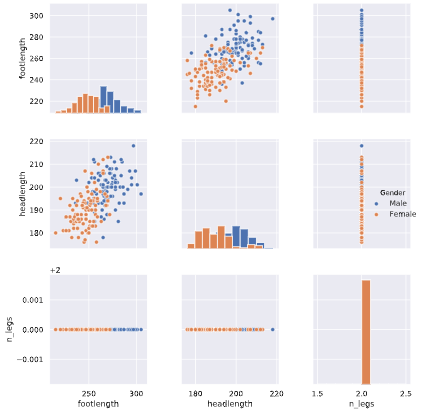One feature has no variance, remove it from the dataset.

``````# Remove the redundant feature
reduced_df = ansur_df_2.drop('n_legs', axis=1)

# Create a pairplot and color the points using the 'Gender' feature
sns.pairplot(reduced_df, hue='Gender', diag_kind='hist')
# Show the plot
plt.show()
``````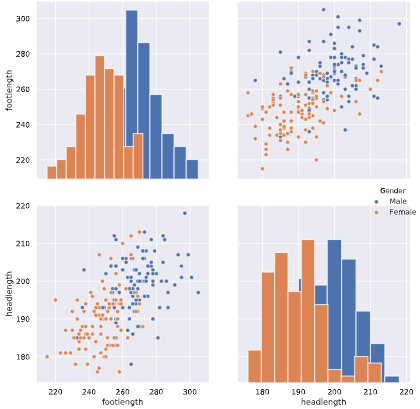The body height (inches) and stature (meters) hold the same information in a different unit + all the individuals in the second sample have two legs.